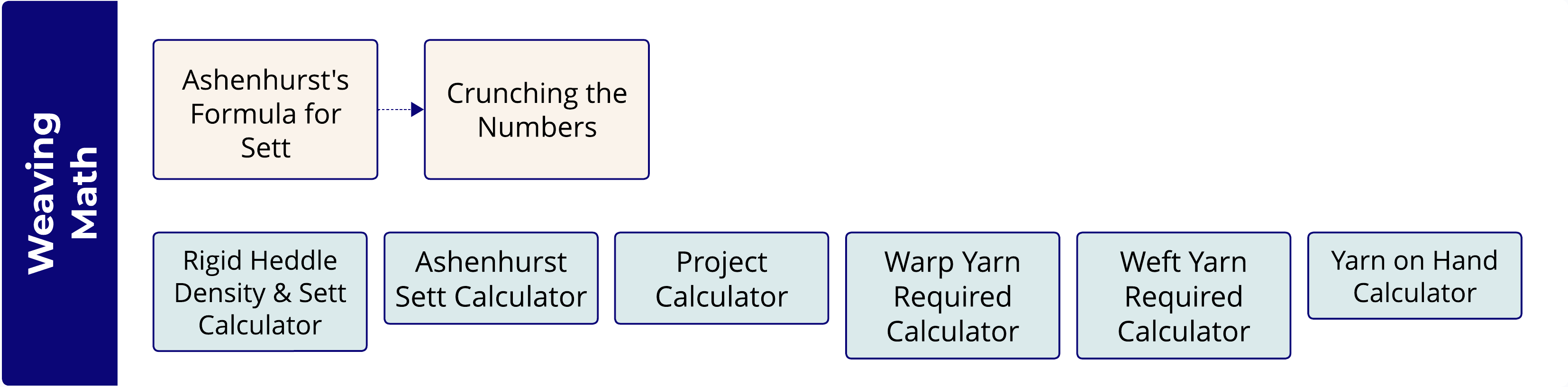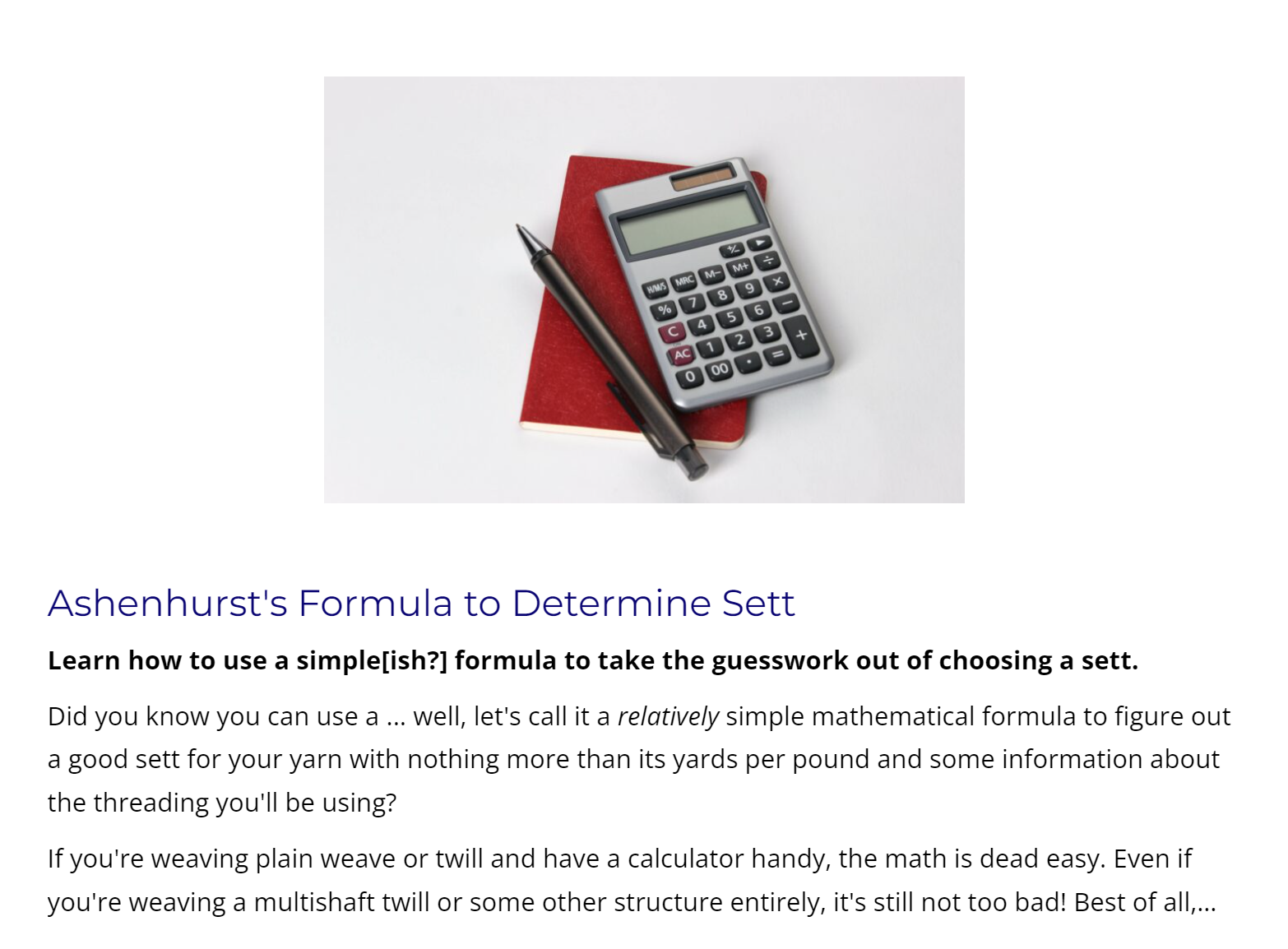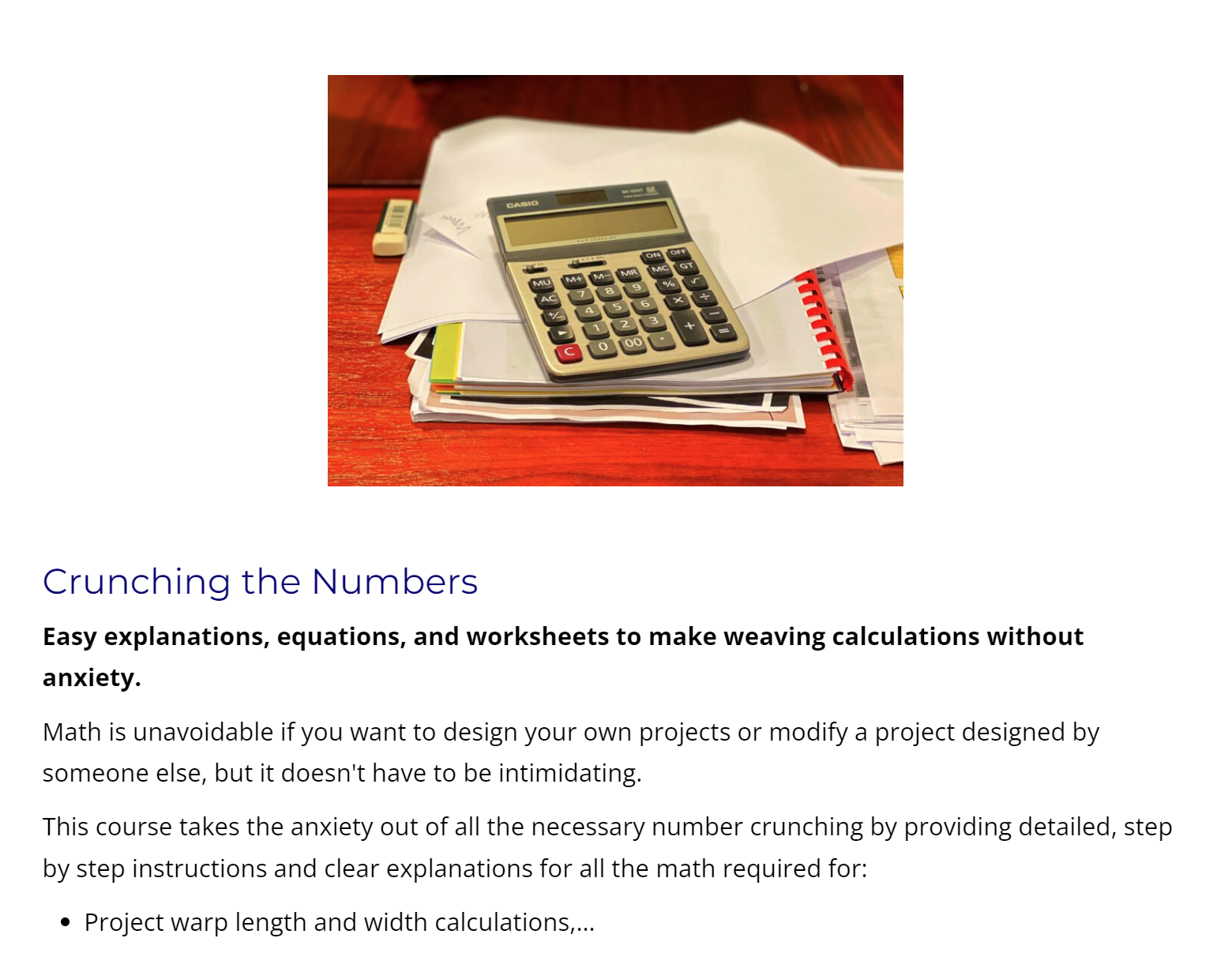## Ashenhurst's Formula for Sett## Crunching the Numbers## Ashenhurst Sett Calculator

The Sett Calculator uses Ashenhurst's formulae to calculate the maximum possible sett for a particular yarn in a given structure and then recommends an appropriate sett for several qualities of cloth.

## Project Calculator

Our Weaving Project Calculator helps you crunch all the numbers for your next project in one convenient spot.

## Warp Yarn Required Calculator

Calculate the amount of warp yarn needed for a project.

## Weft Yarn Required Calculator

Calculate the amount of weft yarn needed for a project.

## Yarn on Hand Calculator

Calculate the amount of yarn you have available based on its weight.